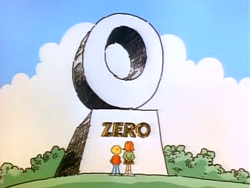# What is 0^0? And is math true, or just useful?

Julia Galef in Measure of Doubt:When you hear mathematicians talk about “searching” for a proof or having “discovered” a new theorem, the implication is that math is something that exists out there in the world, like nature, and that we gradually learn more about it. In other words, mathematical questions are objectively true or false, independent of us, and it’s up to us to discover the answer. That’s a very popular way to think about math, and a very intuitive one.

The alternate view, however, is that math is something we invent, and that math has the form it does because we decided that form would be useful to us, not because we discovered it to be true. Skeptical? Consider imaginary numbers: The square root of X is the number which, when you square it, yields X. And there’s no real number which, when you square it, yields -1. But mathematicians realized centuries ago that it would be useful to be able to use square roots of negative numbers in their formulas, so they decided to define an imaginary number, “i,” to mean “the square root of -1.” So this seems like a clear example in which a mathematical concept was invented, rather than discovered, and in which our system of math has a certain form simply because we decided it would be useful to define it that way, not because that’s how things “really are.”

More here.